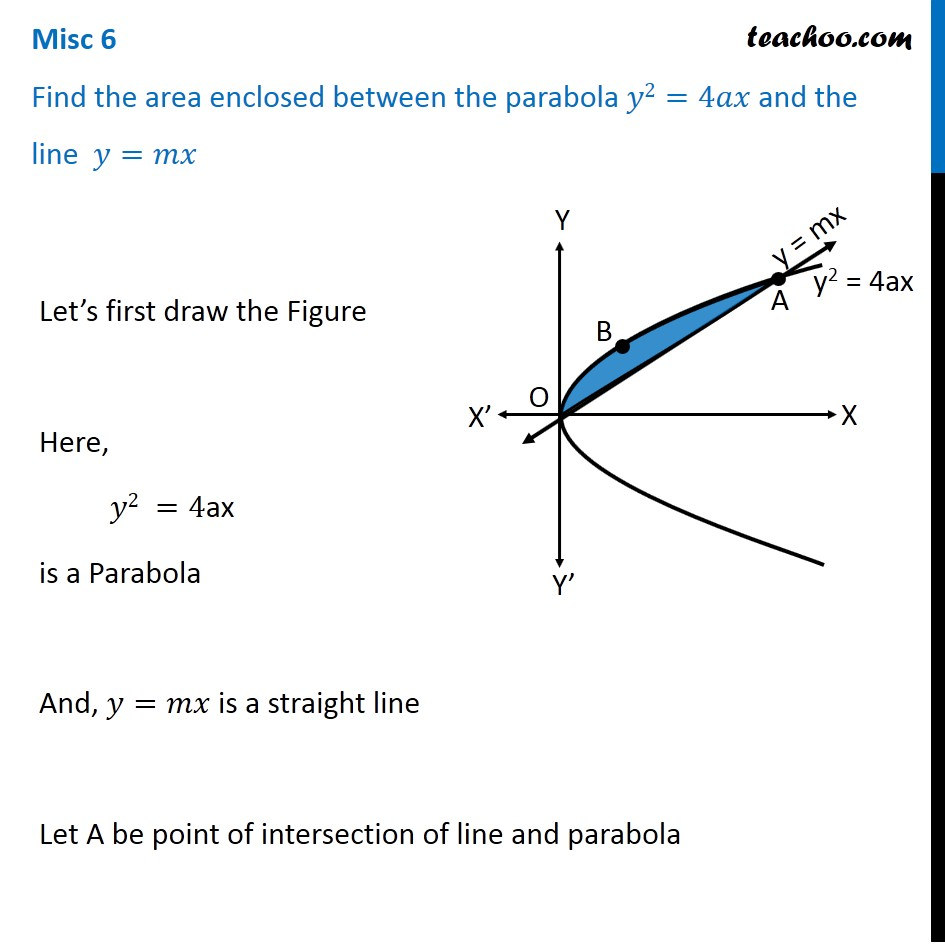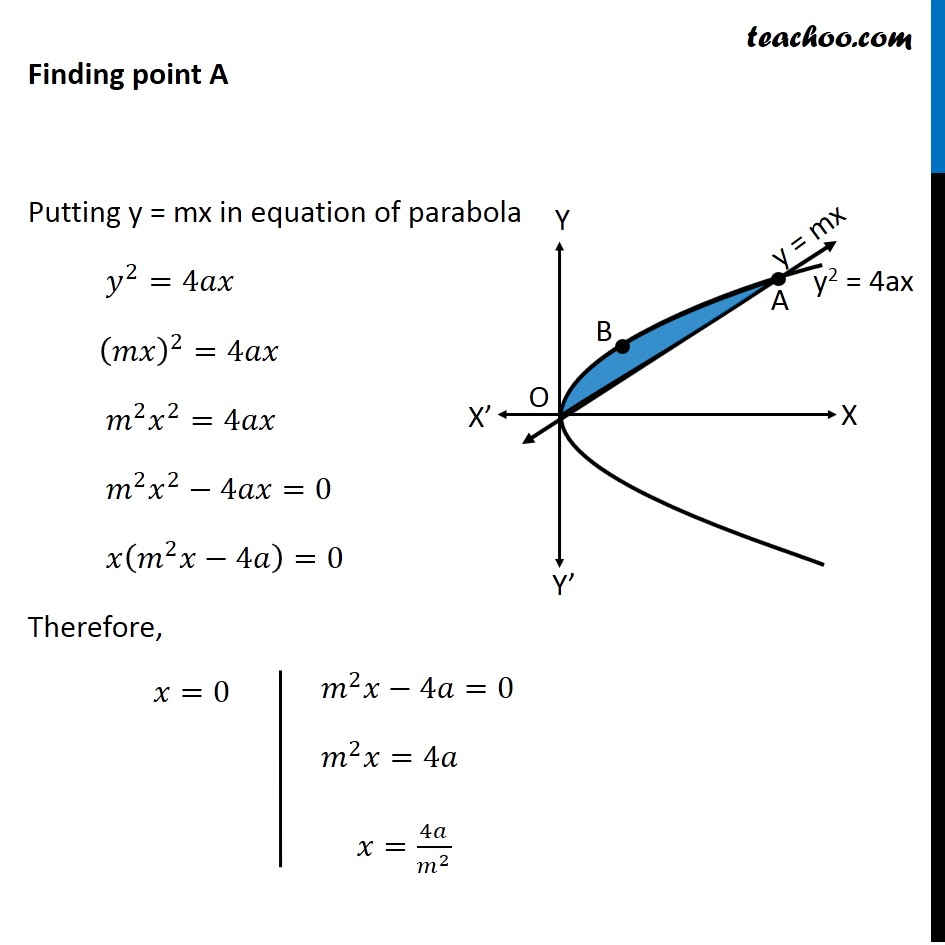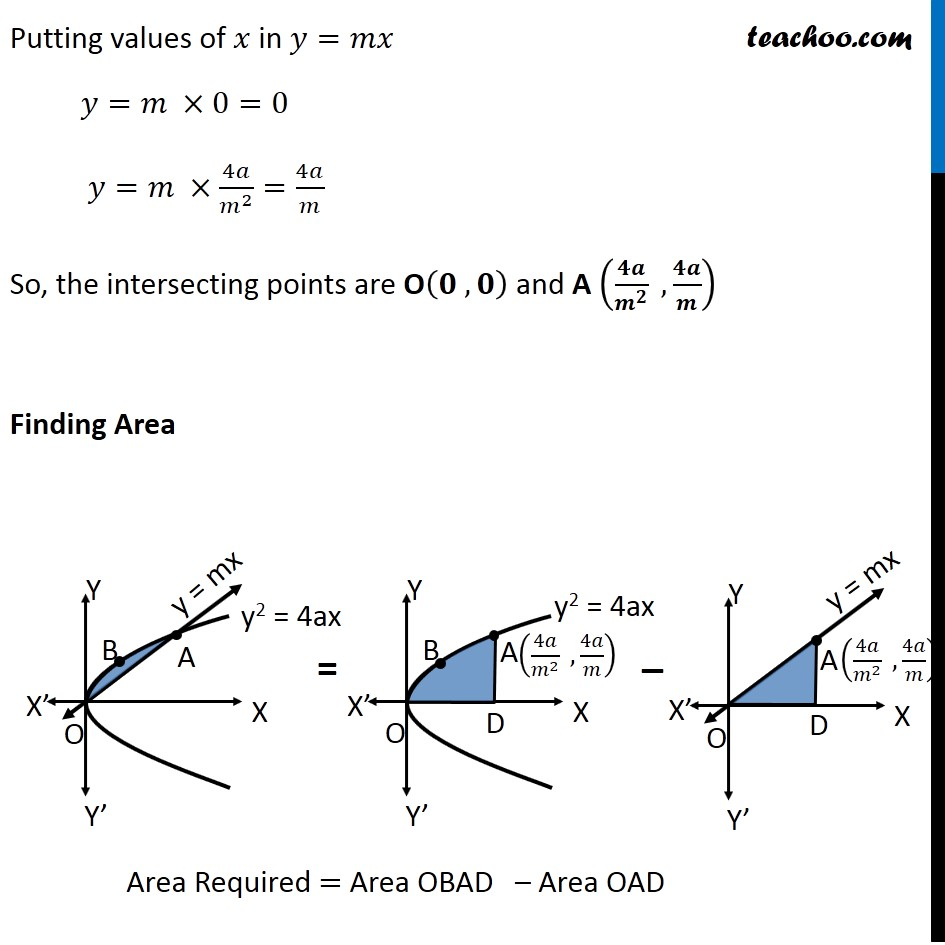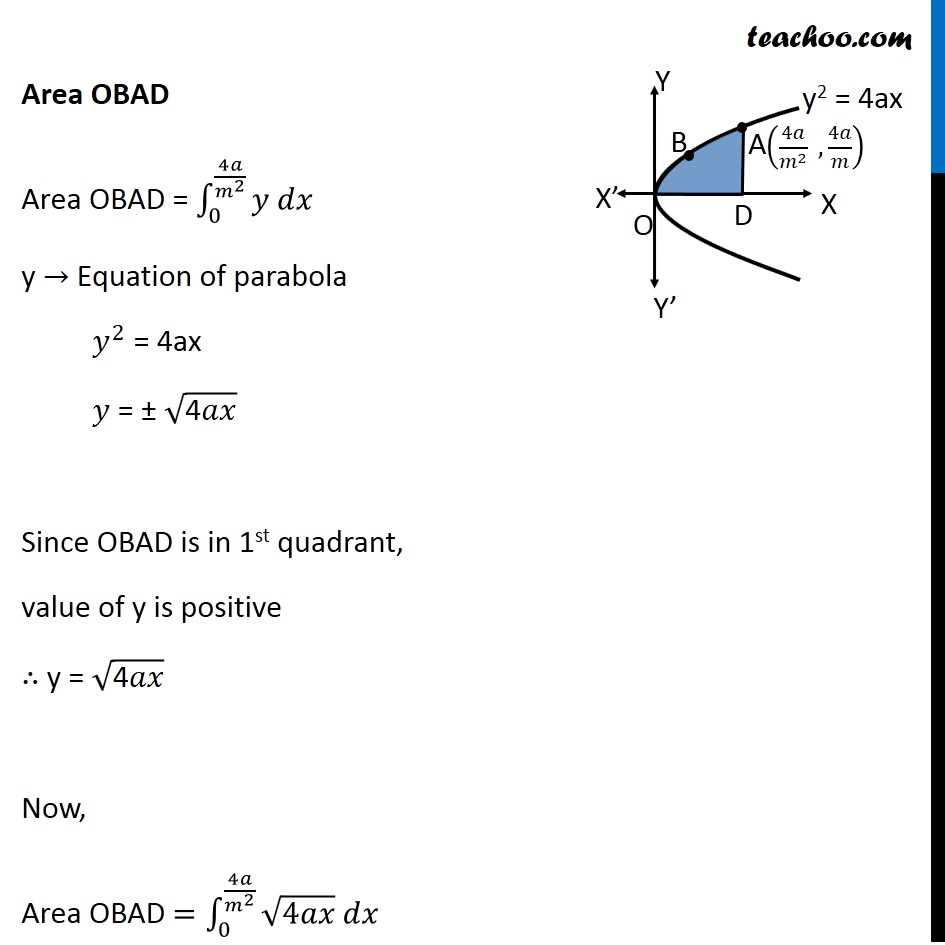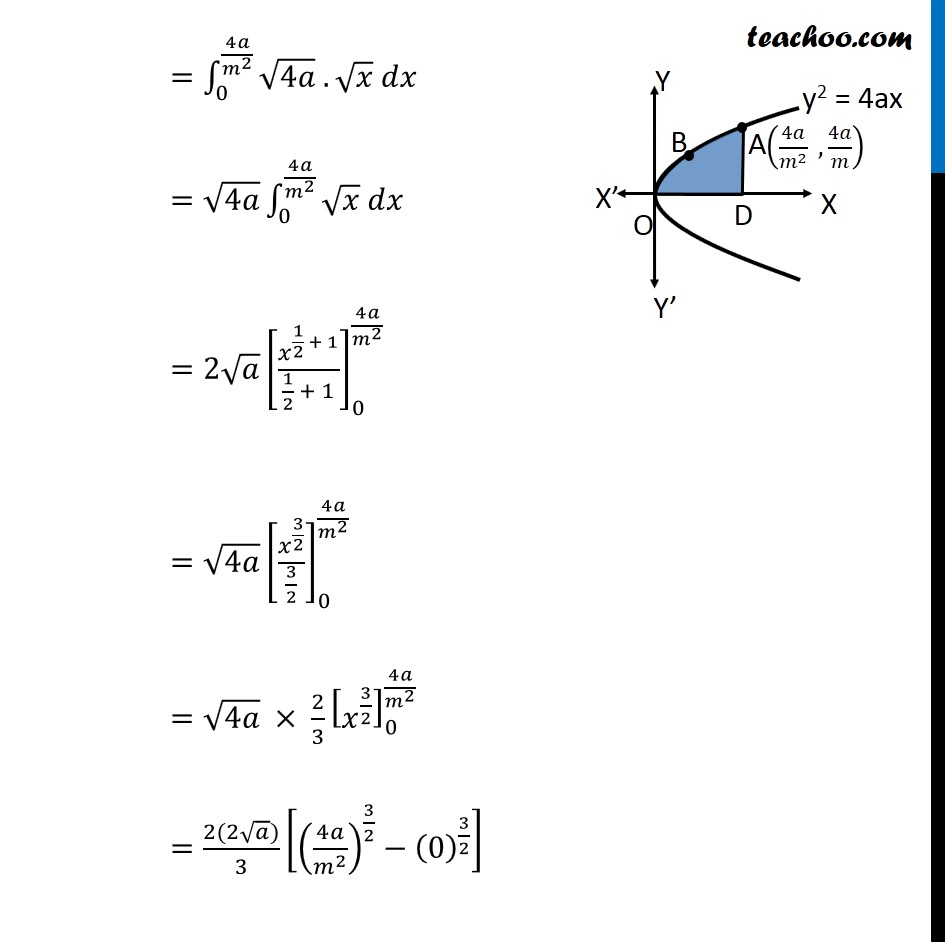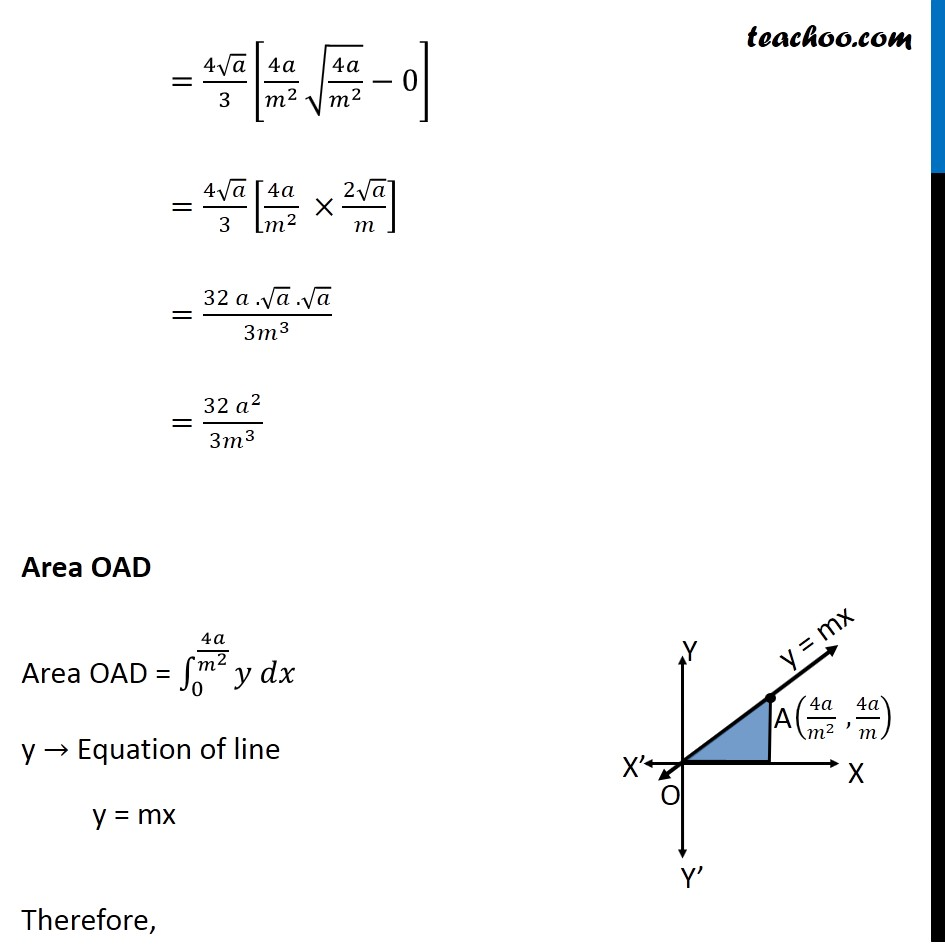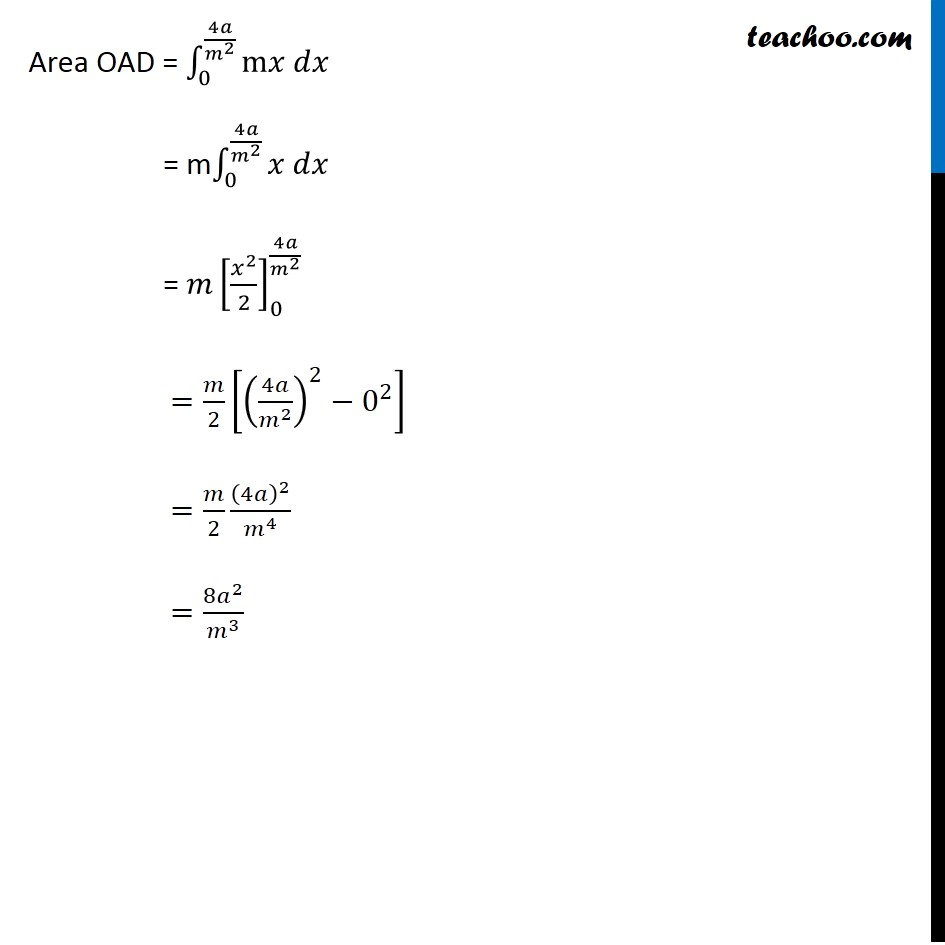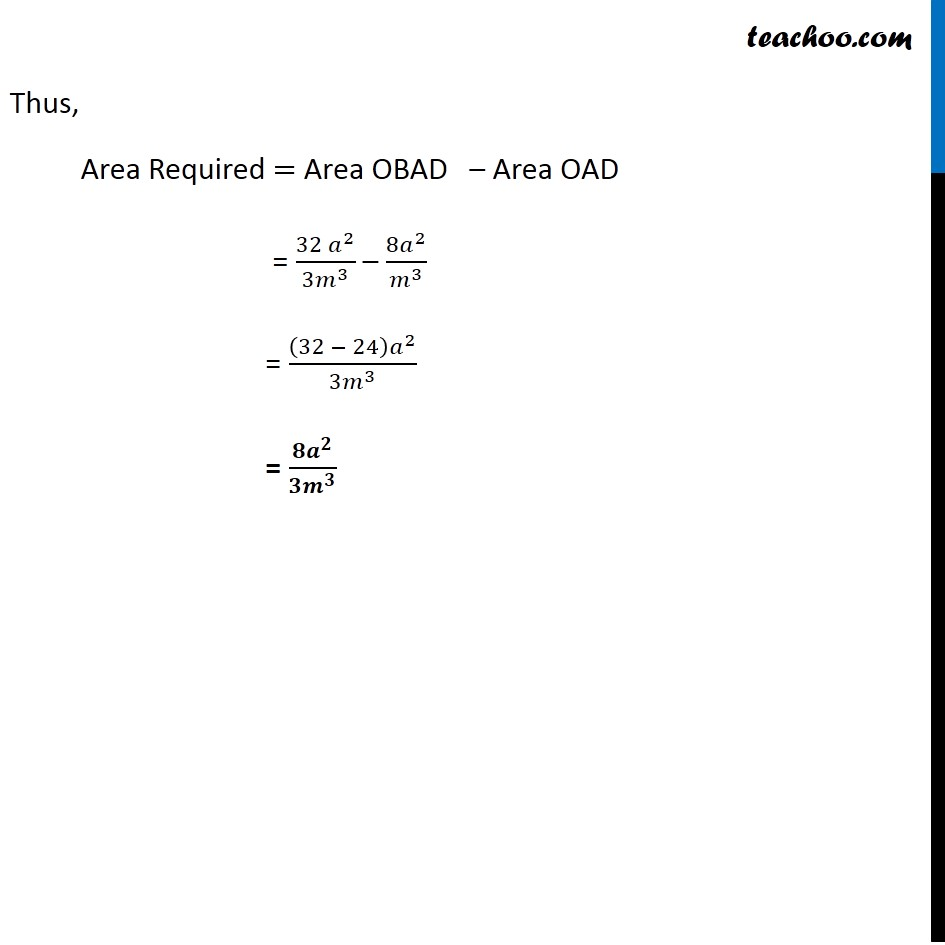1. Chapter 8 Class 12 Application of Integrals
2. Serial order wise
3. Miscellaneous

Transcript

Misc 6 Find the area enclosed between the parabola 𝑦2=4𝑎𝑥 and the line 𝑦=𝑚𝑥 Let’s first draw the Figure Here, 𝑦2 =4ax is a Parabola And, 𝑦=𝑚𝑥 is a straight line Let A be point of intersection of line and parabola Finding point A Putting y = mx in equation of parabola 𝑦^2=4𝑎𝑥 (𝑚𝑥)^2=4𝑎𝑥 𝑚^2 𝑥^2=4𝑎𝑥 𝑚^2 𝑥^2−4𝑎𝑥=0 𝑥(𝑚^2 𝑥−4𝑎)=0 Therefore, 𝑥=0 𝑚^2 𝑥−4𝑎=0 𝑚^2 𝑥=4𝑎 𝑥=4𝑎/𝑚^2 Putting values of 𝑥 in 𝑦=𝑚𝑥 𝑦=𝑚 ×0=0 𝑦=𝑚 ×4𝑎/𝑚^2 =4𝑎/𝑚 So, the intersecting points are O(𝟎 , 𝟎) and A (𝟒𝒂/𝒎^𝟐 ,𝟒𝒂/𝒎) Finding Area Area Required = Area OBAD – Area OAD Area OBAD Area OBAD = ∫_0^(4𝑎/𝑚^2 )▒〖𝑦 𝑑𝑥" " 〗 y → Equation of parabola 𝑦^2 = 4ax 𝑦 = ± √("4" 𝑎𝑥) Since OBAD is in 1st quadrant, value of y is positive ∴ y = √("4" 𝑎𝑥) Now, Area OBAD =∫_0^(4𝑎/𝑚^2 )▒〖√4𝑎𝑥 𝑑𝑥" " 〗 =∫_0^(4𝑎/𝑚^2 )▒〖√4𝑎 .√𝑥 𝑑𝑥" " 〗 =√4𝑎 ∫_0^(4𝑎/𝑚^2 )▒〖√𝑥 𝑑𝑥" " 〗 =2√𝑎 [𝑥^(1/2 + 1)/(1/2 + 1)]_0^(4𝑎/𝑚^2 ) =√4𝑎 [𝑥^(3/2)/(3/2)]_0^(4𝑎/𝑚^2 ) =√4𝑎 × 2/3 [𝑥^(3/2) ]_0^(4𝑎/𝑚^2 ) =(2(2√𝑎))/3 [(4𝑎/𝑚^2 )^(3/2)−(0)^(3/2) ] =(4√𝑎)/3 [4𝑎/𝑚^2 √(4𝑎/𝑚^2 )−0] =(4√𝑎)/3 [4𝑎/𝑚^2 ×(2√𝑎)/𝑚] =(32 𝑎 .√𝑎 .√𝑎)/(3𝑚^3 ) =(32 𝑎^2)/(3𝑚^3 ) Area OAD Area OAD = ∫1_0^(4𝑎/𝑚^2 )▒〖𝑦 𝑑𝑥〗 y → Equation of line y = mx Therefore, Area OAD = ∫1_0^(4𝑎/𝑚^2 )▒〖"m" 𝑥 𝑑𝑥〗 = m∫1_0^(4𝑎/𝑚^2 )▒〖𝑥 𝑑𝑥〗 = 𝑚[𝑥^2/2]_0^(4𝑎/𝑚^2 ) = 𝑚[𝑥^2/2]_0^(4𝑎/𝑚^2 ) =𝑚/2 [(4𝑎/𝑚^2 )^2−0^2 ] =𝑚/2 (4𝑎)^2/𝑚^4 =(8𝑎^2)/𝑚^3 Thus, Area Required = Area OBAD – Area OAD = (32 𝑎^2)/(3𝑚^3 ) – (8𝑎^2)/𝑚^3 = ((32 − 24) 𝑎^2)/(3𝑚^3 ) = (𝟖𝒂^𝟐)/〖𝟑𝒎〗^𝟑

Miscellaneous

About the AuthorDavneet Singh
Davneet Singh is a graduate from Indian Institute of Technology, Kanpur. He has been teaching from the past 10 years. He provides courses for Maths and Science at Teachoo.